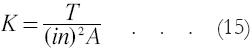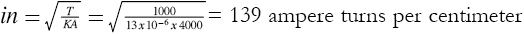# The Thrust Given a Projectile by an Electric Gun

(Reproduced from "Zero to Eighty" pp.294-297 by EF Northrup)

Let us suppose that we have a projectile held stationary in a series of coils (as shown in figure 7, page 309) and acted upon by a travelling magnetic field. We shall now consider the important question: under stated conditions, with what force, i.e., with what thrust, will the magnetic field strive to move the projectile in the direction in which it travels? A purely mathematical solution is theoretically possible but very complicated. We shall, therefore, resort to properly devised tests by which we may determine the value of the so-called thrust constant. When this constant is experimentally determined for a combination of coils and a projectile of one size under particular conditions, the above question becomes comparatively easy to answer for other dimensions and conditions. Several careful tests have been made and the thrust constant, K, has been experimentally determined with a fair precision. In all cases the magnitude of the total thrust is a function of several variables which the designer has power to control.

In what follows it is assumed that no magnetic material is used in the construction of the coils or the projectile.

* * * * *

The starting point for most investigations into electromagnetism and electromagnetic induction is the ampere turn. The terms ampere turns or ampere turns per centimeter are convenient ways of expressing magnetizing force in easily determined and recognized units. The total magnetizing force, in ampere turns, of a coil or helix of wire is simply the product of the number of physically parallel and adjacent conductors and the current in amperes flowing in each conductor. For convenience, it is assumed that the parallel conductors of the helix are one continuous spirally wound conductor, and that the same quantity of electricity flows through the conductor from end to end.

It can be shown that the intensity of the magnetizing force in a closely wound helix is a function of the number of ampere turns per centimeter of winding length of the helix. Since the expressions for fundamental units of magnetic force and magnetic intensity contain terms involving mechanical force, we find that under certain conditions an ampere turn is capable of producing a certain mechanical force.

One such condition is that above stated, namely, a conducting cylinder held stationary in a series of coils carrying high-frequency polyphase currents.

The experimental procedure for getting the data for calculating a thrust constant is as follows: Given a certain set of coils and a cylinder, the thrust in grams on the cylinder, with a certain number of amperes flowing in each phase, is taken for a number of different frequencies. If a sufficient number of frequencies are tried it will be found that one particular frequency gives the highest value of thrust in proportion to the value of the polyphase current. This peak value of thrust is used to calculate the peak thrust constant for the type of polyphase coil used. Other sets of polyphase coils having different proportions among the factors of coil length, coil spacing, size of conducting cylinders, etc., would require the same experimental treatment to find the peak value of thrust.

Having determined the peak value of thrust for the different sets of coils and cylinders, we first define the thrust constant as the force in grams which one ampere turn per centimeter of polyphase current dll impart to one square centimeter of active surface on the cylinder. Secondly, the thrust constant as defined is calculated by the following formula:where K is the thrust constant in grams per square centimeter of active cylinder surface, T the total measured thrust in grams on the cylinder, (in) the average ampere turns per centimeter length of complete polyphase coil, and A the active area in square centimeters of the cylinder, i.e., the product of the circumference of the cylinder and the length covered by the polyphase coil.

Having found the thrust constant, K, for a particularly proportioned test, we can arrange equation (15) to find any one of the factors when the others are known or selected. For instance, a certain type of polyphase coil gave a peak thrust constant of approximately 13 x 10-6 grams. The active area of the test cylinder was about 4000 square centimeters. What value of ampere turns per centimeter length would impart a total thrust of one kilogram to the cylinder?

Arranging equation (15) for ampere turns,If the coil winding averaged one turn per centimeter, the polyphase current per phase would be 139 amperes.

The variable over which the designer has the most control is the number of ampere turns on the coil. Certain features of practical construction limit the actual number of conductors on a coil, but the value of the current in these conductors can be varied over wide limits. The limiting factors are voltage breakdown and heating, the effects of which can be greatly modified by suitable choice of materials and construction.

It may be said that, given a polyphase coil and cylinder, there is at least a theoretical value of current which will produce any desired thrust. This last statement has an important bearing other than its bearing on the question of theoretically producing unlimited thrust. It has been stated above that with a particular polyphase coil and cylinder only one frequency will produce the peak value of the thrust constant. (This assumes that no magnetic materials were used in the construction of coil or cylinder.) Thus, under normal conditions — with a projectile in motion under the influence of a polyphase magnetic field — the thrust constant departs from its maximum value, and hence an increased value of current or ampere turns is necessary to produce the desired thrust.

There is no simple accurate formula which will give the thrust constant for a given set-up at any other than the optimum frequency. Were the factors of resistance and reactance of the various current paths simple proportionate quantities, a formula could be set up. However, alternating current phenomena are mathematically very complex, and in this case do not lend themselves to any simple treatment. For design purposes, it is therefore best to determine experimentally the curve which the thrust constant takes under varying frequency. Enough experimental work has been done along this line to fix a number of points for extrapolating a reasonable curve.

 < Previous Page 4 of 17 Next >